# Tamilnadu Board Class 10 Maths Solutions Chapter 2 Numbers and Sequences Ex 2.10

## Tamilnadu State Board Class 10 Maths Solutions Chapter 2 Numbers and Sequences Ex 2.10

Multiple choice questions

Question 1.
Euclid’s division lemma states that for positive integers a and b, there exist unique integers q and r such that a = bq + r, where r must satisfy.
(1) 1 < r < b
(2) 0 < r < b
(3) 0 < r < b
(4) 0 < r < b
(3) 0 < r < b

Question 2.
Using Euclid’s division lemma, if the cube of any positive integer is divided by 9 then the possible remainders are
(1) 0,1,8
(2) 1,4,8
(3) 0,1,3
(4) 1,3,5
(1) 0,1,8
Hint:
Cube of any +ve integers 13, 23, 33, 43,. . .
1,8,27,64,125,216 …
Remainders when 27, 64, 125 are divided by 9.

Question 3.
If the H.C.F of 65 and 117 is expressible in the form of 65m – 117 , then the value of m is
(1) 4
(2) 2
(3) 1
(4) 3
(2) 2
Hint:
H.C.F. of 65 and 117
117 = 65 × 1 + 52
65 = 52 × 1 + 13
52 = 13 × 4 + 0
∴ 13 is the H.C.F. of 65 and 117.
65m – 117 = 65 × 2 – 117
130 – 117 = 13
∴ m = 2

Question 4.
The sum of the exponents of the prime factors in the prime factorization of 1729 is
(1) 1
(2) 2
(3) 3
(4) 4
(3) 3
Hint:
1729 = 71 × 131 × 191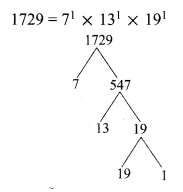Question 5.
The least number that is divisible by all the numbers from 1 to 10 (both inclusive) is
(1) 2025
(2) 5220
(3) 5025
(4) 2520
(4) 2520
Hint: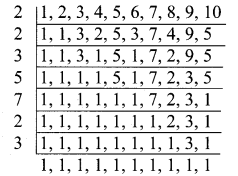∴ L.C.M. of 1,2,3,4,…,10 is 2 × 2 × 3 × 5 × 7 × 2 × 3 = 2520

Question 6.
74k ≡ ______ (mod 100)
(1) 1
(2) 2
(3) 3
(4) 4
(1) 1
Hint:
74k ≡______ (mod 100)
y4k ≡ y4 × 1 = 1 (mod 100)

Question 7.
Given F1 = 1, F2 = 3 and Fn = Fn-1 + Fn-2 then
(1) 3
(2) 5
(3) 8
(4) 11
(4) 11
F1 = 1, F2 = 3
Fn = Fn-1 + Fn-2
F5 = F5-1 + F5-2 = F4 + F3
= F3 + F2 + F2 + F1
= F2 + F1 + F2 + F2 + F1
= 3 + 1 + 3 + 3 + 1 = 11

Question 8.
The first term of an arithmetic progression is unity and the common difference is 4. Which of the following will be a term of this A.P
(1) 4551
(2) 10091
(3) 7881
(4) 13531
(3) 7881
Hint:
t1 = 1
d = 4
tn = a + (n – 1)d
= 1 + 4n – 4
4n – 3 = 4551
4n = 4554
n = will be a fraction
It is not possible.
4n – 3 = 10091
4n = 10091 + 3 = 10094
n = a fraction
4n – 3 = 7881
4n = 7881 + 3 = 7884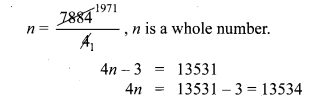n is a fraction.
∴ 7881 will be 1971th term of A.P.

Question 9.
If 6 times of 6th term of an A.P is equal to 7 times the 7th term, then the 13th term of the A.P. is
(1) 0
(2) 6
(3) 7
(4) 13
(1) 0
Hint:
6t6 = 7t7
6(a + 5d) = 7(a + 6d)
6a + 30d = 7a + 42d
7a + 42d – 6a – 30d = 0
a + 12d = 0 = t13

Question 10.
An A.P consists of 31 terms. If its 16th term is m, then the sum of all the terms of this A.P. is
(1) 16 m
(2) 62 m
(3) 31 m
(4) $$\frac { 31 }{ 2 }$$ m
(3) 31 m
Hint:
t16 = m
S31 = $$\frac { 31 }{ 2 }$$ (2a + 30d)
= $$\frac { 31 }{ 2 }$$ (2(a + 15d))
(∵ t16 = a + 15d)
= 31(t16) = 31m

Question 11.
In an A.P., the first term is 1 and the common difference is 4. How many terms of the A.P must be taken for their sum to be equal to 120?
(1) 6
(2) 7
(3) 8
(4) 9
(3) 8
Hint: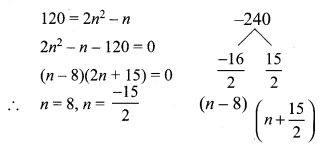Question 12.
If A = 265 and B = 264 + 263 + 262 + +20 which of the following is true?
(1) B is 264 more than A
(2) A and B are equal
(3) B is larger than A by 1
(4) A is larger than B by 1
(4) A is larger than B by 1
Hint:
A = 265
B = 264 + 263 + 262 + … + 20
B = 20 + 21 + 22 + … + 264
G.P = 1 + 21 + 22 + … + 264 it is a G.P
Here a = 1, r = 2, n = 65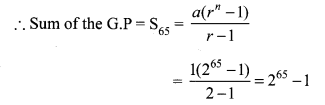A = 265, B = 265 – 1
∴ B is smaller.
A is larger than B by 1.

Question 13.
The next term of the sequence $$\frac { 3 }{ 16 }$$,$$\frac { 1 }{ 8 }$$,$$\frac { 1 }{ 12 }$$,$$\frac { 1 }{ 18 }$$, ….
(1) $$\frac { 1 }{ 24 }$$
(2) $$\frac { 1 }{ 27 }$$
(3) $$\frac { 2 }{ 3 }$$
(4) $$\frac { 1 }{ 81 }$$
(2) $$\frac { 1 }{ 27 }$$
Hint: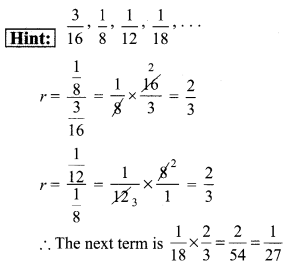Question 14.
If the sequence t1,t2,t3 … are in A.P. then the sequence t6,t12,t18 … is
(1) a Geometric Progression
(2) an Arithmetic Progression
(3) neither an Arithmetic Progression nor a Geometric Progression
(4) a constant sequence
(2) an Arithmetic Progression
Hint:
If t1, t2, t3, … is 1, 2, 3, …
If t6 = 6, t12 = 12, t18 = 18 then 6, 12, 18 … is an arithmetic progression

Question 15.
The value of (13 + 23 + 33 + … + 153) – (1 + 2 + 3 + … + 15) is
(1) 14400
(2) 14200
(3) 14280
(4) 14520